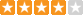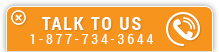# Liquid Pipeline Hydraulics

Course Number: O-5002
Credit: 5 PDH
Subject Matter Expert: E. Shashi Menon, P.E.
Price: \$149.75 Purchase using Reward Tokens. Details34 reviews
Overview

#### In Liquid Pipeline Hydraulics , you'll learn ...

• Properties of liquids
• Calculating Reynolds Number and friction factor
• How to calculate liquid pressure drop in a pipeline using Moody Diagrams and pressure drop equations such as Miller Equation, MIT Equation and Hazen Williams
• Components of total pressure and how to maintain the hydraulic pressure gradient above the elevation profile

#### OverviewPreview a portion of this course before purchasing it.

Credit: 5 PDH

Length: 74 pages

This online course on liquid pipeline hydraulics covers the steady state transportation of liquids in pipelines. These include water lines, refined petroleum products and crude oil pipelines. This course will prove to be a refresher in fluid mechanics as it is applied to real world pipeline design. Although many formulas and equations are introduced, we will concentrate on how these are applied to the solution of actual pipeline transportation problems.

First, the liquid properties are discussed and how they vary with temperature and pressure are analyzed. The pressure in a liquid and liquid head are explained next. Then the classical Darcy equation for determining pressure drop due to friction in liquid flow is introduced and a modified more practical version is explained. Common forms of equations relating flow versus pressure drop due to friction are introduced and applications illustrated by example problems. In a long distance pipeline the need for multiple pump stations and hydraulic pressure gradient are discussed.

Next the pumping horsepower required to transport a liquid through a pipeline is calculated. Centrifugal and positive displacement pumps are discussed along with an analysis of the pump performance curves. The impact of liquid specific gravity and viscosity on pump performance is explained with reference to the Hydraulic Institute charts. The use of drag reduction as a means to improving pipeline throughput is explored. Batching of different products in a pipeline simultaneously, with minimum commingling, is discussed next. The internal design pressure in a pipeline and the hydrostatic test pressure for safe operation are explained with illustrative examples.

#### Specific Knowledge or Skill Obtained

This course teaches the following specific knowledge and skills:

• Properties of liquids
• Calculating Reynolds Number and friction factor
• How to calculate liquid pressure drop in a pipeline using Moody Diagrams and pressure drop equations such as Miller Equation, MIT Equation and Hazen Williams
• Components of total pressure and how to maintain the hydraulic pressure gradient above the elevation profile
• The effect of temperature on liquid pipeline calculations
• Looping a pipeline to increase flow rate
• Determining the quantity and location of pumping stations and the required horsepower
• Plotting pump vs. system head curves
• Using the pump affinity laws
• Evaluating pump curves, including head vs. flow rate, efficiency vs. flow rate, BHP vs. flow rate, NSPH vs. flow rate
• Using drag reduction agents (DRA) to increase a pipeline's capacity
• Pumping different products, such as jet fuel and diesel, through a pipeline using batching methods
• Calculating pipe wall thickness based on MAOP

#### Certificate of Completion

You will be able to immediately print a certificate of completion after passing a multiple-choice quiz consisting of 25 questions. PDH credits are not awarded until the course is completed and quiz is passed.

Board Acceptance
 This course is applicable to professional engineers in: Alabama (P.E.) Alaska (P.E.) Arkansas (P.E.) Delaware (P.E.) Florida (P.E. Area of Practice) Georgia (P.E.) Idaho (P.E.) Illinois (P.E.) Illinois (S.E.) Indiana (P.E.) Iowa (P.E.) Kansas (P.E.) Kentucky (P.E.) Louisiana (P.E.) Maine (P.E.) Maryland (P.E.) Michigan (P.E.) Minnesota (P.E.) Mississippi (P.E.) Missouri (P.E.) Montana (P.E.) Nebraska (P.E.) Nevada (P.E.) New Hampshire (P.E.) New Jersey (P.E.) New Mexico (P.E.) New York (P.E.) North Carolina (P.E.) North Dakota (P.E.) Ohio (P.E. Self-Paced) Oklahoma (P.E.) Oregon (P.E.) Pennsylvania (P.E.) South Carolina (P.E.) South Dakota (P.E.) Tennessee (P.E.) Texas (P.E.) Utah (P.E.) Vermont (P.E.) Virginia (P.E.) West Virginia (P.E.) Wisconsin (P.E.) Wyoming (P.E.)
Reviews (34)
More DetailsPreview a portion of this course before purchasing it.

Credit: 5 PDH

Length: 74 pages